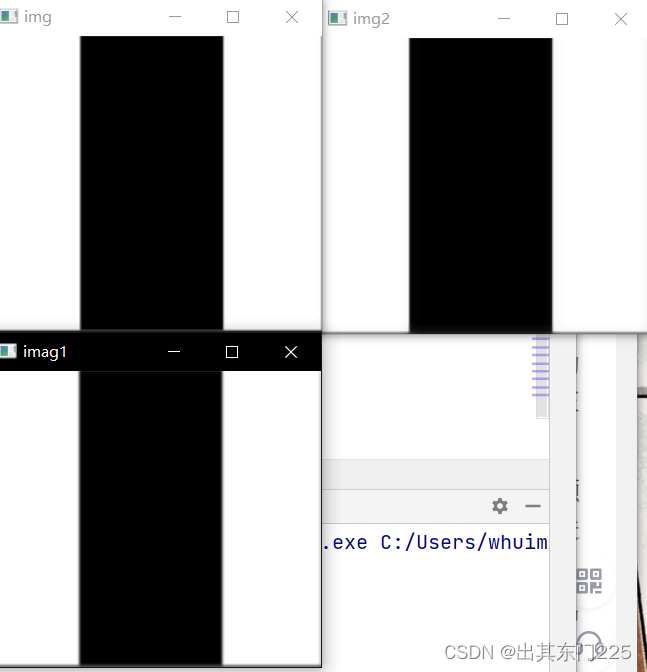# 模板匹配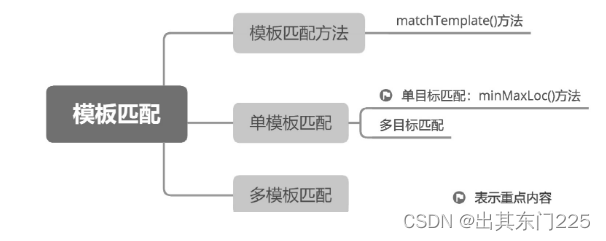## 模板匹配方法

`` result = cv2.matchTemplate(image, templ, method, mask)``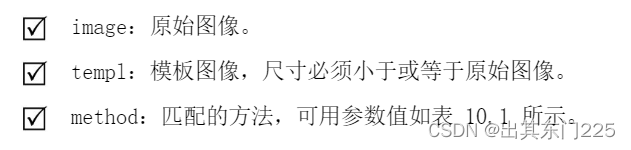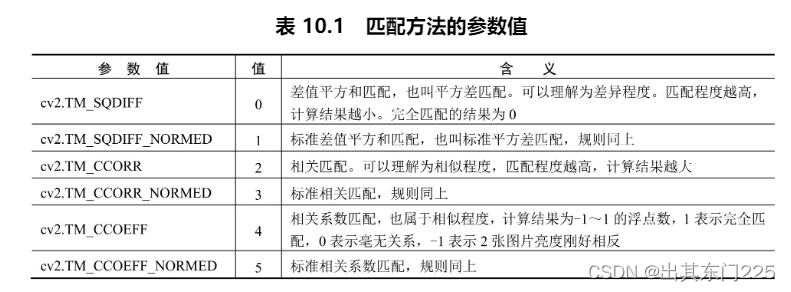result：计算得出的匹配结果。如果原始图像的宽、高分别为W、H，模板图像的宽、高分别为w、h，result 就是一个W-w+1 列、H-h+1 行的 32 位浮点型数组。数组中每一个浮点数都是原始图像中对应像素位置的匹配结果，其含义需要根据 method 参数来解读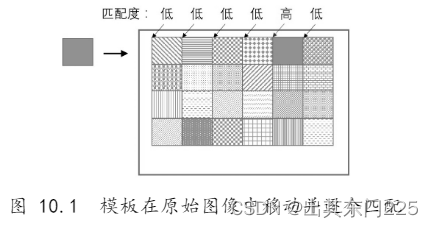### 单目标匹配

matchTemplate()方法的计算结果是一个二维数组，OpenCV 提供了一个 minMaxLoc()方法专门用来解析这个二维数组中的最大值、最小值以及这 2 个值对应的坐标，minMaxLoc()方法的语法如下：

``minValue, maxValue, minLoc, maxLoc = cv2.minMaxLoc(src, mask)``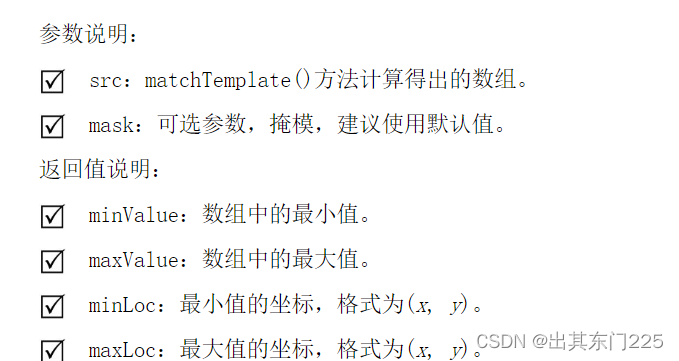``````import cv2
import numpy as np
temple=cv2.resize(temple,(45,45))
cv2.imshow('t',temple)
result=cv2.matchTemplate(img,temple,cv2.TM_SQDIFF_NORMED)
minvalue,maxvalue,minloc,maxloc=cv2.minMaxLoc(result)
height,width,t=temple.shape
point1=minloc
point2=(point1+width,point1+height)
cv2.rectangle(img,point1,point2,(0,0,255),3)
cv2.imshow('img',img)
cv2.waitKey()
cv2.destroyAllWindows()
cv2.waitKey(0)
cv2.destroyAllWindows()``````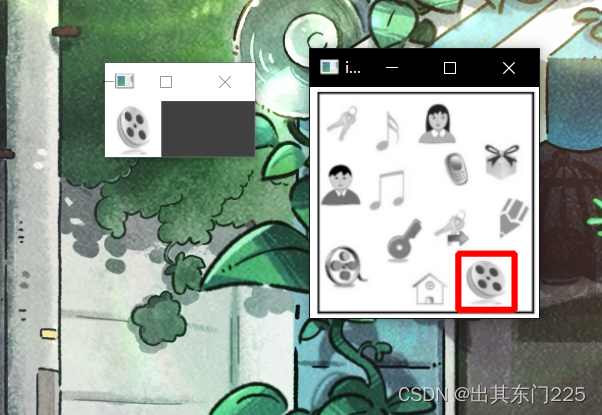### 多目标匹配# 滤波器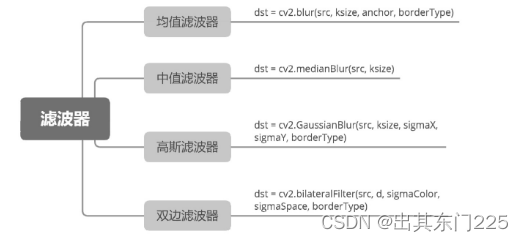### 均值滤波器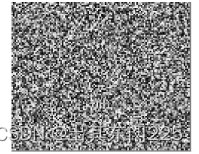例如，图 11.4 就是均值滤波的计算过程。滤波核大小为 3×3，核心像素值是 35，颜色较深，周围像素值都为 110～150，因此可以认为核心像素是噪声。将滤波核中的所有像素值相加，然后除以像素个数，就得出了平均值 123（四舍五入取整）。将核心像素的值改成 123，其颜色就与周围颜色差别不大，图像就变得平滑了。这就是均值滤波去噪的原理。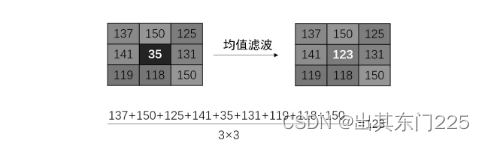OpenCV 将均值滤波器封装成 blur()方法，其语法如下：

``  dst = cv2.blur(src, ksize, anchor, borderType)``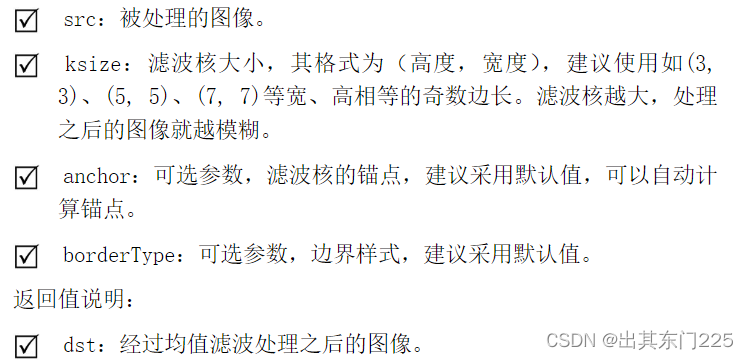``````import  cv2
import numpy as np
dst=cv2.blur(img,(3,3))
dst2=cv2.blur(img,(9,9))
cv2.imshow('dst2',dst2)
cv2.imshow('img',img)
cv2.imshow('sdt',dst)
cv2.waitKey(0)
cv2.destroyAllWindows()``````

### 中值滤波器

OpenCV 将中值滤波器封装成 medianBlur()方法，其语法如下：

`  dst = cv2.medianBlur(src, ksize)`

``````import cv2
import numpy as np
image1=cv2.medianBlur(image,3)
image2=cv2.medianBlur(image,5)
image3=cv2.medianBlur(image,9)
cv2.imshow('image',image)
cv2.imshow('3',image1)
cv2.imshow('5',image2)
cv2.imshow('9',image3)
cv2.waitKey(0)
cv2.destroyAllWindows()``````### 高斯滤波器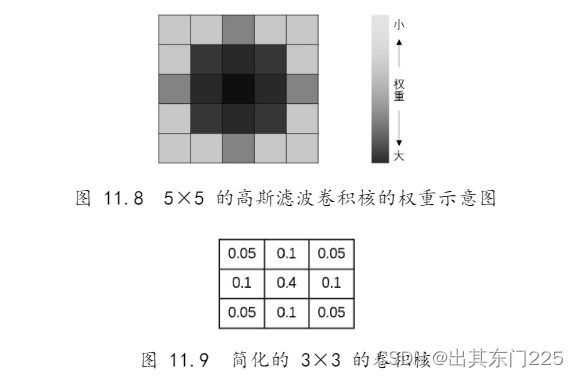OpenCV 将高斯滤波器封装成了 GaussianBlur()方法，其语法如下：

`  dst = cv2.GaussianBlur(src, ksize, sigmaX, sigmaY, borderType)`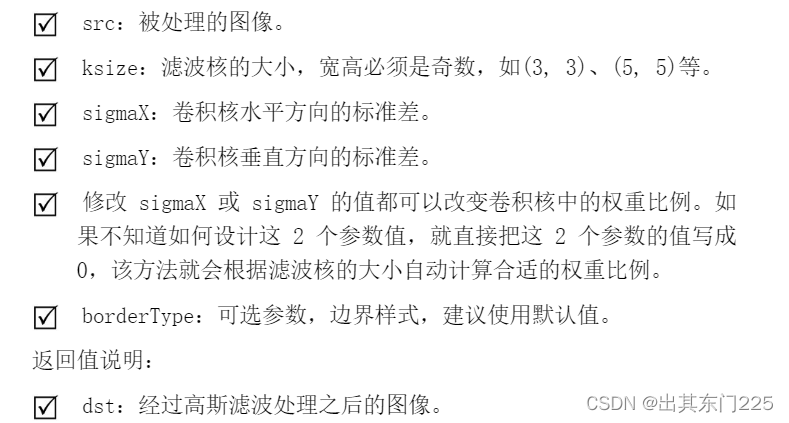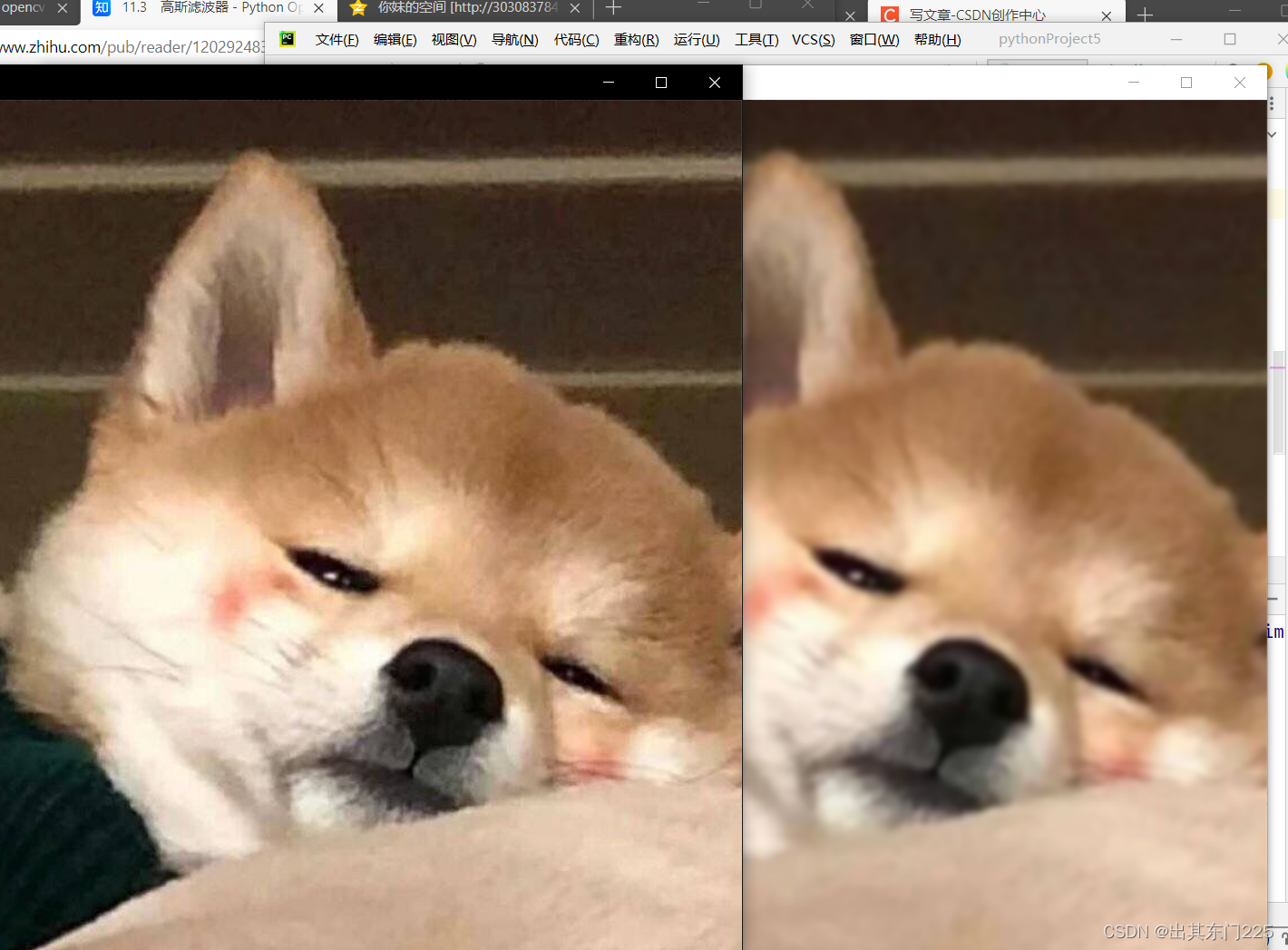``````import  cv2
import numpy as  np
dst1=cv2.GaussianBlur(image,(15,15),0,0)
cv2.imshow('image',image)
cv2.imshow('dst',dst1)
cv2.waitKey(0)
cv2.destroyAllWindows()``````

### 双边滤波器

OpenCV 将双边滤波器封装成 bilateralFilter()方法，其语法如下：

`dst = cv2.bilateralFilter(src, d, sigmaColor, sigmaSpace, borderType)`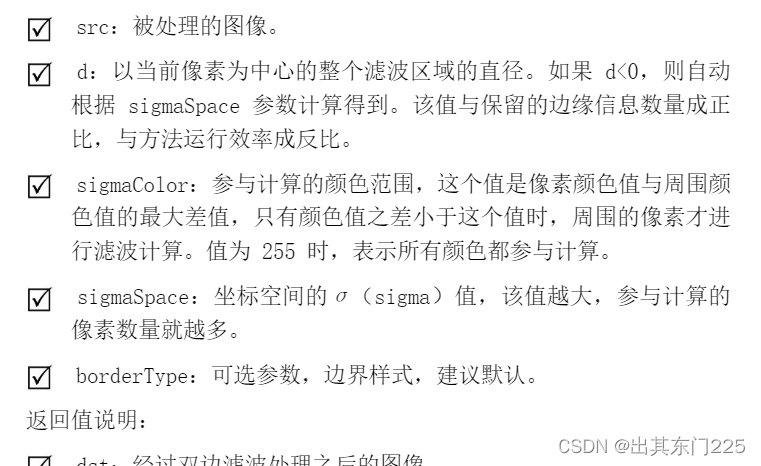``````import cv2
import numpy as  np